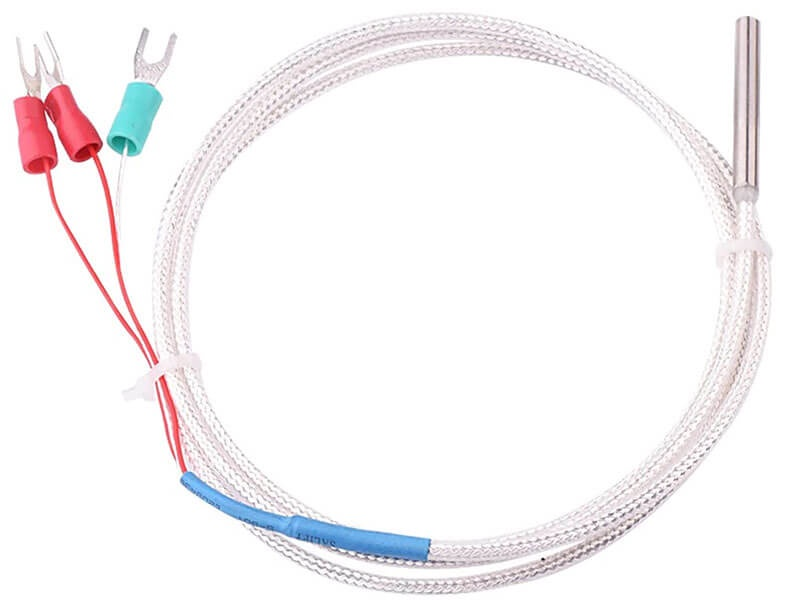## Platinum RTD Temperature Sensor PT100

SGD12.00
••••SGD12.00

## DESCRIPTION

Platinum resistance temperature detectors (RTDs) offer excellent accuracy over a wide temperature range (from –200 to +850 °C). Standard sensors are are available from many manufacturers with various accuracy specifications and numerous packaging options to suit most applications. Unlike thermocouples, it is not necessary to use special cables to connect to the sensor.

The principle of operation is to measure the resistance of a platinum element. The most common type (PT100) has a resistance of 100 ohms at 0 °C and 138.4 ohms at 100 °C. There are also PT1000 sensors that have a resistance of 1000 ohms at 0 °C.

The relationship between temperature and resistance is approximately linear over a small temperature range: for example, if you assume that it is linear over the 0 to 100 °C range, the error at 50 °C is 0.4 °C. For precision measurement, it is necessary to linearise the resistance to give an accurate temperature. The most recent definition of the relationship between resistance and temperature is International Temperature Standard 90 (ITS-90).

The linearization equation is:

Rt = R0 * (1 + A* t + B*t2 + C*(t-100)* t3)

Where:

Rt is the resistance at temperature t, R0 is the resistance at 0 °C, and
A= 3.9083 E–3
B = –5.775 E–7
C = –4.183 E–12 (below 0 °C), or
C = 0 (above 0 °C)

For a PT100 sensor, a 1 °C temperature change will cause a 0.384 ohm change in resistance, so even a small error in measurement of the resistance (for example, the resistance of the wires leading to the sensor) can cause a large error in the measurement of the temperature. For precision work, sensors have four wires- two to carry the sense current, and two to measure the voltage across the sensor element. It is also possible to obtain three-wire sensors, although these operate on the (not necessarily valid) assumption that the resistance of each of the three wires is the same.

## TECHNICAL DETAILS

• Operating Range: -200 to +550°C
• Accuracy: ±0.5°C from -10 to +85°C
• Probe Length: 40mm
• Probe Diameter: 4mm
• Cable Length: 1m
• Dimensions: 18 x 18 x 75mm
• Weight: 16g